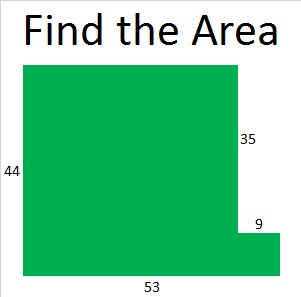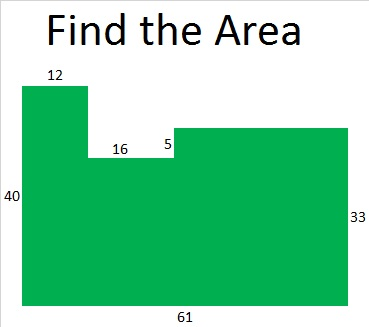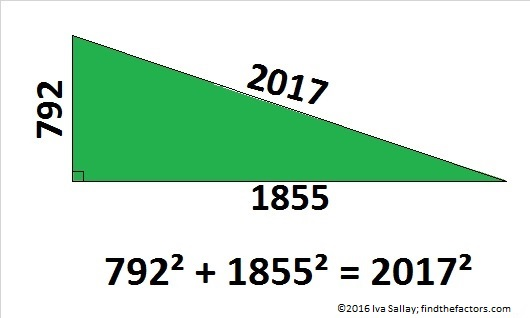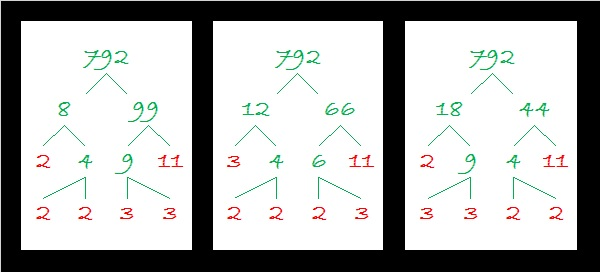# 792 Number Facts and Factors of the Year 2017

Happy New Year, everybody!

So much of what I’ve included in this post is a bit difficult for elementary school students, but here is an area problem that they should be able to do:Perhaps you’ll recognize that as just another way to illustrate that 44² + 9² = 2017.

This area problem based on 33² + 28² + 12² = 2017 will be a little bit more challenging for students:2016 had more factors, positive and negative, than anybody could have imagined, but 2017 is a prime number year, so hopefully it will be filled with less drama.

I have a lot to say about the number 2017 with a little help from twitter.

2017 has only TWO positive real factors, 1 and 2017, and only TWO negative real factors, -1 and -2017. Positive or negative, ALL the real possible factor pairs for 2017 are

• 1 x 2017 and (-1) x (-2017).

2017 = 4(504) + 1. So 2017 is the sum of two squares. Which ones?

44² + 9² = 2017. That sum-of-squares number fact means that 2017 is the hypotenuse of a Pythagorean triple, specifically, 792-1855-2017. Since 2017 is a prime number, this triple is also a primitive.Here’s how those numbers were calculated from the fact that  44² + 9² = 2017:

• 2(44)(9) = 792
• 44² – 9² = 1855
• 44² + 9² = 2017

2017 is also the short leg in a rather monstrous primitive Pythagorean triple:

• 2017² + 2,034,144² = 2,034,145²

Hmm. 44² + 9² = 2017 means we can find some COMPLEX factor pairs for 2017:

• (44 + 9i)(44 – 9i) = 2017
• (-44 + 9i)(-44 – 9i) = 2017
• (9 + 44i)(9 – 44i) = 2017
• (-9 + 44i)(-9 – 44i) = 2017

Who knows what all those COMPLEX factors will bring to the coming year? Each of them was derived from the fact that 44² + 9² = 2017.

2017 is the sum of THREE squares three different ways:

• 37² + 18² + 18²  = 2017
• 33² + 28² + 12² = 2017
• 30² + 26² + 21² = 2017

Since 37² = 35² + 12², 30² = 24² + 18², and 26² = 24² + 10², we can write 2017 as the sum of these squares, too:

• 35² + 18² + 18² + 12² = 2017
• 30² + 24² + 21² + 10² = 2017
• 26² + 24² + 21² + 18² = 2017
• 24² + 24² + 21² + 18² + 10² = 2017

Here’s more sums and/or differences of squares from twitter:

This representation of 2017 is “two” powerful:

2017 is a PALINDROME in bases 31, 32, and 36:

• 232 BASE 31; note that 2(31²) + 3(31) + 2(1) = 2017
• 1V1 BASE 32 (V is 31 base 10); note that 1(32²) + 31(32) + 1(1) = 2017
• 1K1 BASE 36 (K is 20 base 10); note that 1(36²) + 20(36) + 1(1) = 2017

Also Stetson.edu tells us this 2017 fact about the totient function: “2017 is a value of n for which φ(n) = φ(n-1) + φ(n-2).”

PROOF that 2017 is a prime number:

We can use these three facts to help us verify that 2017 is a prime number:

1. 2017 = 4(504) + 1
2. 44² + 9² = 2017
3. √2017 ≈ 44.8998886

Since 2017 can be written as one more than a multiple of four, and 44 and 9 have no common prime factors, 2017 will be a prime number unless it is divisible by 5, 13, 17, 29, 37, or 41 (all the prime numbers less than √2017 ≈ 44.9 that have a remainder of one when divided by 4). That’s right, we ONLY have to divide 2017 by those SIX numbers to verify that it is prime: (Read here for why this is true.)

• 2017 ÷ 5 = 403.4
• 2017 ÷ 13 ≈ 155.15
• 2017 ÷ 17 ≈ 118.65
• 2017 ÷ 29 ≈ 69.55
• 2017 ÷ 37 ≈ 54.51
• 2017 ÷ 41 ≈ 49.20

We don’t get a whole number answer for any of those divisions, so 2017 is prime!

If you’re looking for more reasons to be interested in the number 2017, read David Radcliffe’s article:

Here are some other lovely mathematical thoughts about 2017 that I found on twitter:

In case you don’t know (and apparently a lot of people don’t know), 0! = 1, so this expression really does equal 2017.

And finally, if you click on this next link, David Mitchell will explain the tessellation of the number 2017.

——————————–

Since this is my 792nd post, I’ll write a little bit about the number 792:

792 has 24 factors so I’ll include a few of its many possible factor trees:Since 792 has so many factors, it is a leg in MANY Pythagorean triples. Here I list some factors that generate PRIMITIVE triples:

• 2(396)(1): 792-156815-156817
• 2(99)(4): 792-9785-9817
• 2(44)(9): 792-1855-2017 (illustrated near the top of this post)
• 2(36)(11): 792-1175-1417

792 is the sum of three squares four different ways including three ways that repeat squares:

• 28² + 2² + 2² = 792
• 26² + 10² + 4² = 792
• 20² + 14² + 14² = 792
• 18² + 18² + 12² = 792

792 is a palindrome in bases 32 and 35:

• OO BASE 32 (O is 24 base 10); note that 24(32) + 24(1) = 792
• MM BASE 35 (M is 22 base 10); note that 22(35) + 22(1) = 792

Finally here is the factoring information for the number 792:

• 792 is a composite number.
• Prime factorization: 792 = 2 x 2 x 2 x 3 x 3 x 11, which can be written 792 = (2^3) x (3^2) x 11
• The exponents in the prime factorization are 3, 2 and 1. Adding one to each and multiplying we get (3 + 1)(2 + 1)(1 + 1) = 4 x 3 x 2 = 24. Therefore 792 has exactly 24 factors.
• Factors of 792: 1, 2, 3, 4, 6, 8, 9, 11, 12, 18, 22, 24, 33, 36, 44, 66, 72, 88, 99, 132, 198, 264, 396, 792
• Factor pairs: 792 = 1 x 792, 2 x 396, 3 x 264, 4 x 198, 6 x 132, 8 x 99, 9 x 88, 11 x 72, 12 x 66, 18 x 44, 22 x 36 or 24 x 33

Taking the factor pair with the largest square number factor, we get √792 = (√36)(√22) = 6√22 ≈ 28.14249.